#### You may also like### At a Glance

The area of a regular pentagon looks about twice as a big as the pentangle star drawn within it. Is it?### Six Discs

Six circular discs are packed in different-shaped boxes so that the discs touch their neighbours and the sides of the box. Can you put the boxes in order according to the areas of their bases?### Equilateral Areas

ABC and DEF are equilateral triangles of side 3 and 4 respectively. Construct an equilateral triangle whose area is the sum of the area of ABC and DEF.

# Of All the Areas

##### Age 14 to 16Challenge Level
Guruvignesh from Hymers College in the UK began looking at equilateral triangles with no tilt:
The triangle with isometric length 2 has an area 4, the triangle with isometric length 3 has an area  of 9 and the triangle with an isometric length of 4 has an area of 16. Therefore, the area of the triangle is the square of the length of the base.

The pattern will comtinue because AF = SF$^2$ (area factor = scale factor squared), and so if we double the side to make another similar shape the area will be quadrupled and so will continue to be the square of the base.

Guruvignesh used a diagram similar to this one to explain why the tilted triangles are equilateral: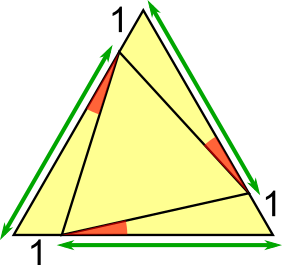If the tilted triangle has a tilt of 1 then it has been rotated along the side of the equilateral triangle by 1 isometric dot. Since the inner triangle is equilateral, the lines labelled in green are also equal, and each of the 3 outer triangles has an angle of 60$^\text o$, we deduce that they are congruent using the side, angle, side rule.
This means that the lengths of the tilted triangle are all equal and that the red angles are all equal. Either of those facts tell us that the tilted triangle is equilateral.Finding the areas of triangles with tilt 1
Guruvignesh used a similar diagram to find the areas of the triangles with tilt 1.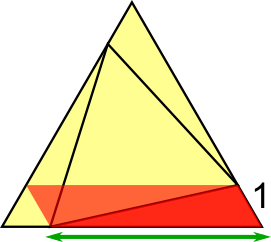The area of the tilted triangle is the outer triangle take away the inner triangles, each of which is half a parallelogram. We know from Isometric Areas that the area of a parallelogram on isometric paper is double the product of the sides.

In the case of the smallest tilted triangle, the side length of the outside equilateral triangle is 3 so the green length is 2. So the area of the parallelogram is 2$\times$1$\times$2 = 4.
Because each triangle has an area of 2, the whole area not taken uo by the tilted triangle is 6 and so the area is 9 $-$ 6, which is 3.

Now, we can find the area of all of the tilted triangles; the green triangle has an area of 7, the blue has an area of 13 and the red has an area of 21.

Matt from Swindon Academy in the UK used paralellograms inside the tilted triangles: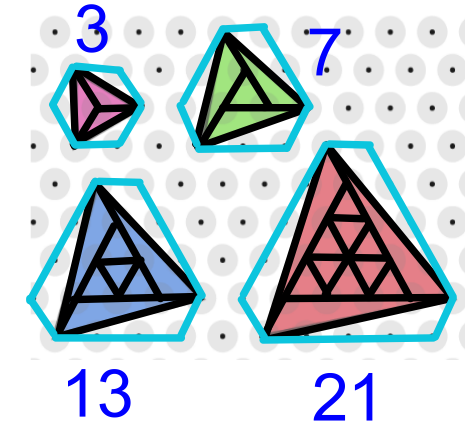By splitting the triangles as shown and considering the halving ratio between triangles and parallelograms and rectangles (that the area of a triangle is half the area of a parallelogram, and we know how to find the area of a parallelogram on isometric paper from Isometric Areas), you can calculate the areas as $(1+1+1),$ $(1+2+2+2),$ $(4+3+3+3),$ $(9+4+4+4).$

The sequence $3,7,13,21,...$ can be solved:
First difference $4,6,8,$ second difference $2$.
$N^2:1,4,9,16$
$N^2+N:2,6,12,20$
$N^2+N+1:3,7,13,21$

Finding areas of triangles with other tilts
Matt went on to find the areas of equilateral triangles with tilt and 3: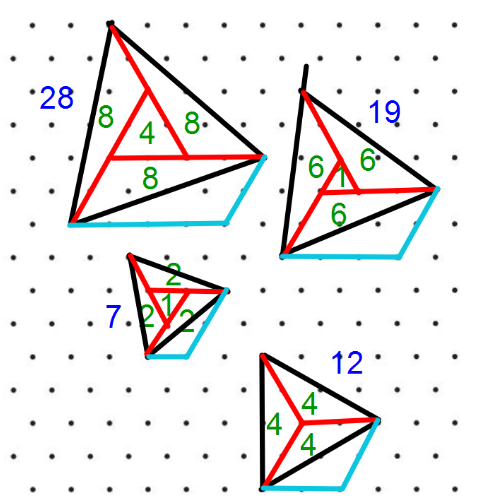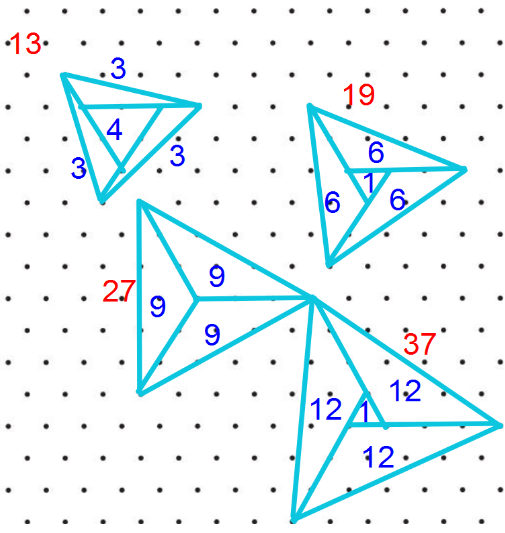Using a similar method, solving the                               Using a similar method, solving the
sequence of a tilt of 2 gives                                           sequence for a tilt of 3 gives
$N^2+2N+4$                                                                 $N^2+3N+9$

This allowed Matt and also Esther to spot a general rule. Matt said:
In general, the rules $N^2+N+1,$ $N^2+2N+4$ and $N^2+3N+9$ all fit the form of $N^2+AN+A^2$, where $A$ is the tilt of the triangle.